# TWSIAM Activity Group : FreeFem++

Contact : Yao-Yu Yang
Yannick Deleuze

# Finite Elements for Fluids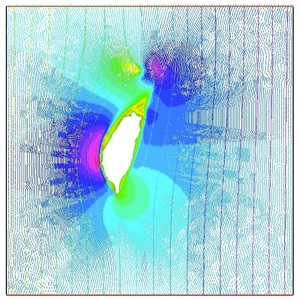## Triangular finite elements

March 10, 2016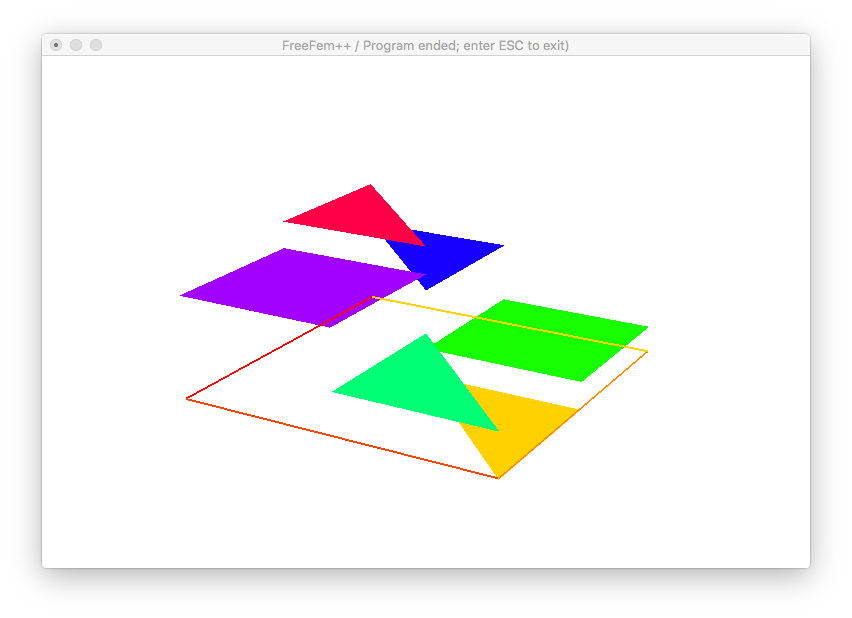Projection of $$f(x,y) = sin(\pi \, x) cos(\pi \, y)$$ on $$\mathrm{Vh(Th,P0)}$$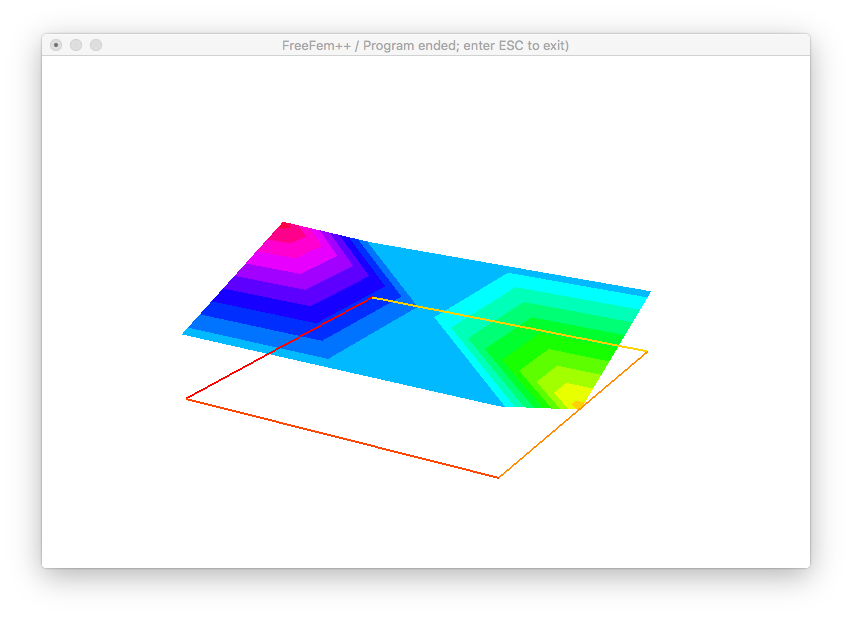Projection of $$f(x,y) = sin(\pi \, x) cos(\pi \, y)$$ on $$\mathrm{Vh(Th,P1)}$$Projection of $$f(x,y) = sin(\pi \, x) cos(\pi \, y)$$ on $$\mathrm{Vh(Th,P2)}$$

Using the files triangles.dat and dof.dat produced by the FreeFem++ script basis.edp, we use GNUplot to exhibit the deegree of freedoms with this command
splot 'triangles.dat' w l notitle, 'dof.dat' t 'Deegrees of freedom' pt 6 lw 3

## Finite Element Mehod in FreeFem++

March 31, 2016

Order of convergence between the computed finite element solution $$u_h$$ and the exact solution $$u$$.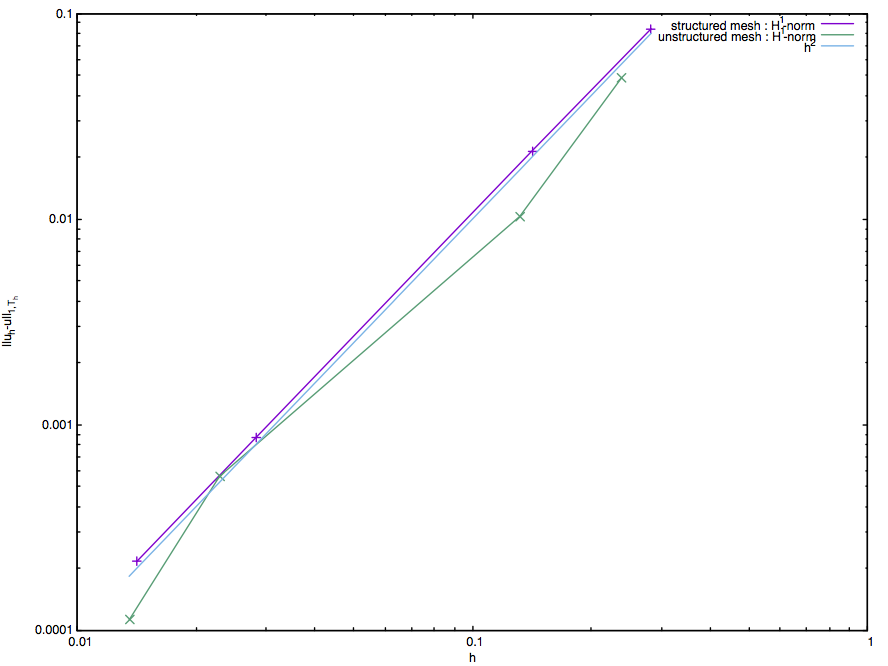P2 finite elements - order 2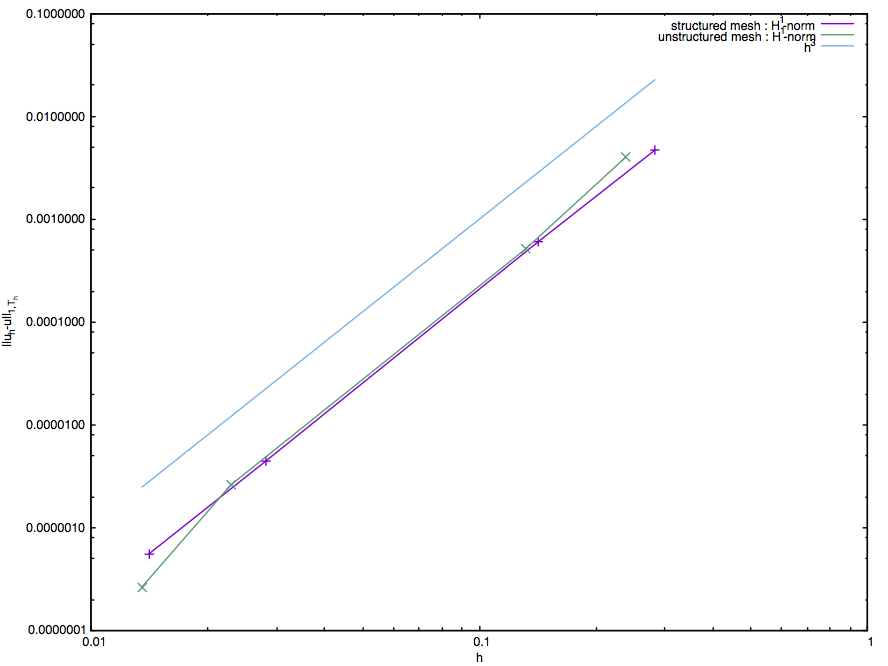P3 finite elements - order 3

Solution $$u_h$$ of the elliptic problem with Robin oundary conditions in $$\Omega=[0,1]×[0,1]$$ :

$$- \nabla^2 u +c\,u= f \text{ in } \Omega$$
$$\frac{\partial u}{\partial n} +\varepsilon u = 0 \text{ on } \partial\Omega$$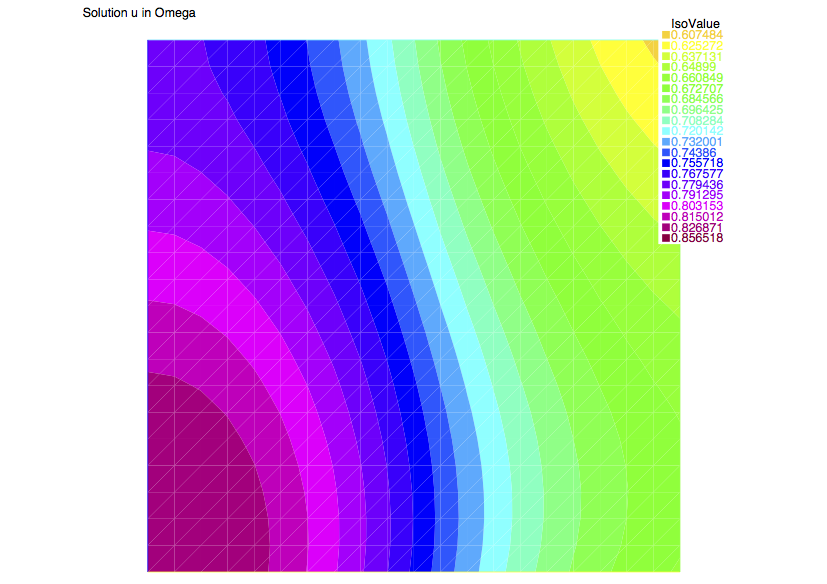$$f = 1$$, $$c = y$$, and $$\varepsilon = 0.1 x$$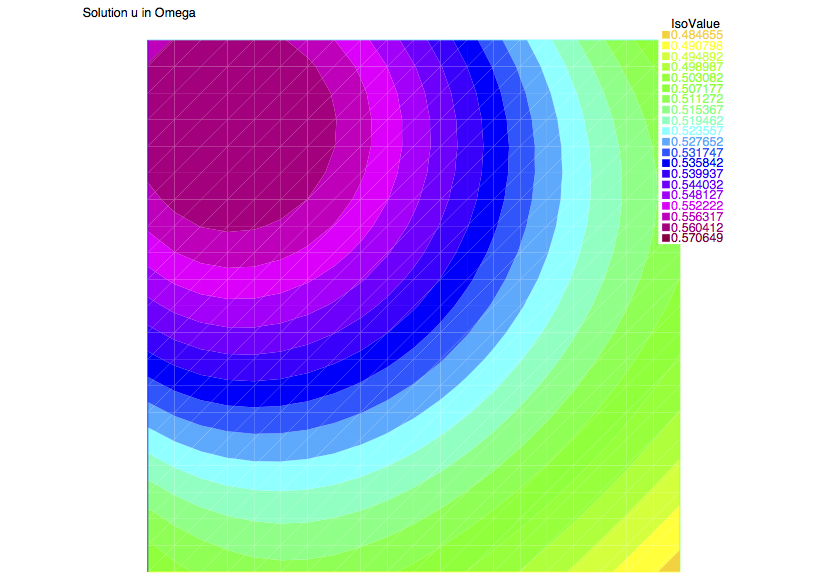$$f=\cos(x)\sin(y)$$, $$c=\min(x,y)$$, and $$\varepsilon =0.1$$

## Convection-diffusion / Stokes equations

April 7, 2016

Convection dominant solution of the convection-diffusion equation in the unit square.Solution along $$x = 0.5$$Solution along $$y = 0.5$$

Solution of the Stokes equations in a vessel with a stenose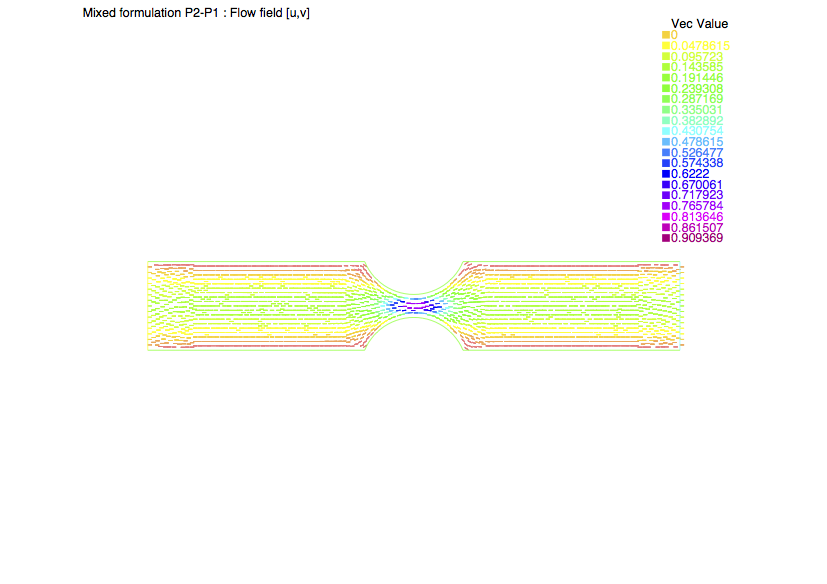$$[u,v]$$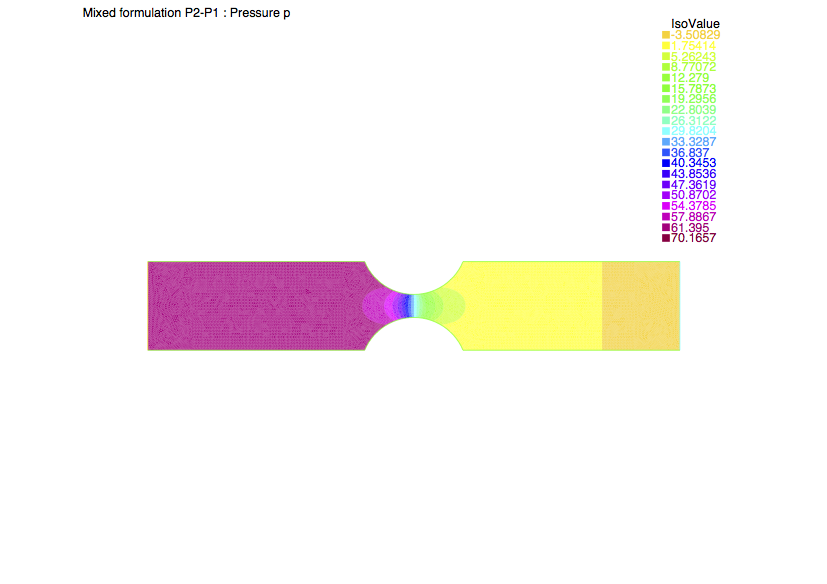$$p$$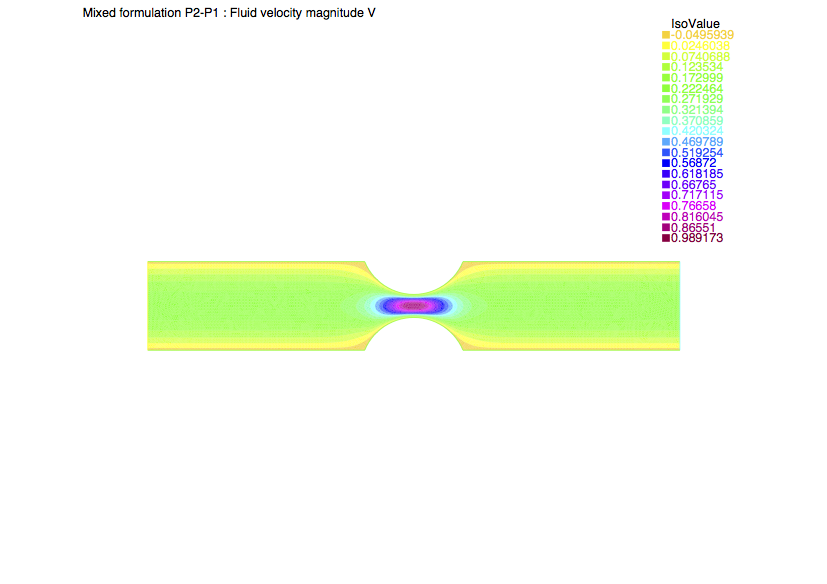$$V = \sqrt{u^2+v^2}$$

May 5, 2016